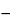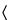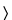Choline Bitartrate

C9H19NO7 253.25
2-Hydroxyethanaminium, -N,N,N-trimethyl-, [R-(R*,R*)]-2,3-dihydroxybutanedioate (1:1);
(2-Hydroxyethyl)trimethylammonium-l-(+)-tartrate salt (1:1)[87-67-2].
DEFINITION
Choline Bitartrate contains NLT 99.0% and NMT 100.5% of choline bitartrate (C9H19NO7), calculated on the anhydrous basis.
IDENTIFICATION
•  B.
Sample:  1 g
Analysis:  Dissolve the Sample in 20 mL of water, and add 2 mL of potassium chloride solution (1 in 4).
Acceptance criteria:  A white precipitate of potassium bitartrate is formed.
ASSAY
•  Procedure
Sample:  200 mg
Titrimetric system
Mode:  Direct titration
Titrant:  0.1 N perchloric acid VS
Endpoint detection:  Potentiometric
Blank:  50 mL of glacial acetic acid
Analysis:  Dissolve the Sample in 50 mL of glacial acetic acid and titrate with Titrant.
Calculate the percentage of choline bitartrate (C9H19NO7) in the Sample taken:
Result = [(VB) × N × F × 100]/W
 V = = Sample titrant volume (mL) B = = Blank titrant volume (mL) N = = titrant normality (mEq/mL) F = = equivalency factor, 253.2 mg/mEq W = = weight of the Sample (mg)
Acceptance criteria:  99.0%–100.5% on the anhydrous basis
IMPURITIES
Meet the requirements, except that the limit for 1,4-dioxane is 10 µg/g
Analysis:  Add 30 mL of water and 5 mL of hydrochloric acid to dissolve the sample.
Acceptance criteria:  NMT 2 ppm
[Note—Use methylene chloride in place of chloroform to prepare the Dithizone Extraction Solution and Standard Dithizone Solution. ]
Solution A:  Transfer 8.4 g of sodium hydroxide solution (1 in 2) to a plastic bottle, add 100 mL of ammonium hydroxide, and mix.
Standard solution:  Transfer 1.0 mL of the Diluted Standard Lead Solution to a separatory funnel containing 25.0 mL of water.
Sample solution:  Dissolve 3.00 g of Choline Bitartrate in a separatory funnel containing 25.0 mL of water.
Analysis
Samples:  Standard solution and Sample solution
Separately add 6.0 mL of Ammonium Citrate Solution and 3.0 mL of Potassium Cyanide Solution to the Standard solution and the Sample solution. Extract each of the resulting solutions three times with 5.0-mL portions of Dithizone Extraction Solution, shaking for 60 s and draining off each extract into another separator. Shake the combined dithizone solutions for 30 s with 20.0 mL of nitric acid (1 in 100), and discard the methylene chloride layer. Add 6.0 mL of Ammonia-Cyanide Solution, 2 mL of Solution A, and 10 mL of Standard Dithizone Solution, and shake for 45 s. Allow the phases to separate, and measure the absorbance of the lower layer at 510 nm with a suitable spectrophotometer.
Acceptance criteria:  The absorbance of the Sample solution is NMT the absorbance of the Standard solution (NMT 0.3 ppm).
•  Limit of Total Amines
Standard solution:  500 µg/mL of trimethylamine hydrochloride
Sample solution:  Transfer 10.0 g of Choline Bitartrate to a beaker containing a plastic-coated stirring bar, add 70 mL of sodium hydroxide TS and 130 mL of water, and stir until dissolved.
System suitability stock solution:  10 µg/mL of trimethylamine hydrochloride
System suitability solution:  Transfer 10.0 mL of System suitability stock solution containing a plastic-coated stirring bar, add 160 mL water and 30.0 mL sodium hydroxide TS, and stir until dissolved.
Electrode system:  Use a gas-sensing, ammonia-specific indicating electrode with internal reference connected to a pH meter capable of measuring potentials with a minimum reproducibility of ±0.1 mV (see pH791).
Standard response line:  Mix 30.0 mL of sodium hydroxide TS and 170 mL of water. Add a plastic-coated stirring bar, insert the electrode into the solution, and record the potential, in mV. Continue stirring, and at 5-min intervals, add 0.200, 0.600, 1.00, and 2.00 mL of Standard solution, and record the potential after each addition. Plot the logarithms of the cumulative trimethylamine hydrochloride concentrations (0.50, 1.50, 2.50, and 5.00 µg/mL) versus potential, in mV, and determine the slope (S) of the Standard response line for the electrode.
System suitability
Sample:  System suitability solution
Proceed as directed in Analysis, except to replace the Sample solution with the System suitability solution and in the formula below to replace W with V, which equals 10 mL.
Suitability requirements:  The total change is NLT 10 mV for a 0.4-mL cumulative addition of the Standard solution; the amount of trimethylamine hydrochloride found is 8.5–11.5 µg/L.
Analysis
Samples:  Standard solution and Sample solution
Rinse the electrode, insert it into the Sample solution, stir, and record the potential, in mV. Add 0.100 mL of the Standard solution, and record the potential. Add another 0.100 mL of the Standard solution, and record the potential. [Note—If the total change after the second addition of the Standard solution is less than 10 mV, add a third aliquot of 0.200 mL. ]
Calculate the content, in µg/g, of total amines as trimethylamine hydrochloride in the portion of sample taken:
Result = (CS × VA)/[(F1) × W]
 CS = = concentration of the Standard solution (µg/mL) VA = = total volume of the Standard solution added to the Sample solution (mL) W = = weight of Choline Bitartrate taken to prepare the Sample solution (g) F = = correction factor, calculated by the formula:
F = antilog [(mVFmV0)/S]
 mVF = = final reading after the additions of the Standard solution (mV) mV0 = = initial reading of the Sample solution (mV) S = = slope of the Standard response line for the electrode
Acceptance criteria:  NMT 10 µg/g
•  Chromatographic Purity
Buffer solution:  7.1 g/L of anhydrous dibasic sodium phosphate. Adjust with phosphoric acid to a pH of 2.5.
Mobile phase:  Buffer solution and acetonitrile (7:3)
Standard solution:  Transfer an amount, NMT 100 mg, of USP Choline Chloride RS to a 24-mL screw-capped vial, and add 400 mg of 3,5-dinitrobenzoyl chloride and 10 mL of acetonitrile. Cap the vial, heat to 55, and continue heating for 2 h. Cool to room temperature, and add 5 mL of water. Allow to stand for 5 min. Quantitatively transfer the solution to a 25-mL volumetric flask and dilute with acetonitrile to volume. Dilute a volume of this solution with Mobile phase to obtain a concentration of 2.0 µg/mL of USP Choline Chloride RS.
Sample solution:  Transfer 500 mg of Choline Bitartrate to a centrifuge tube, add 2.0 mL of water, and swirl to dissolve. Add 0.5 mL of potassium chloride solution (7.5 in 25), centrifuge, and transfer 1.0 mL of the supernatant to a 24-mL screw-capped vial. Dry at 120for 2 h. Add 400 mg of 3,5-dinitrobenzoyl chloride and 10 mL of acetonitrile. Cap the vial, and heat at 55for 2 h. Cool to room temperature, add 5 mL of water, and allow to stand for 5 min. Quantitatively transfer this solution to a 50-mL volumetric flask, and dilute with Mobile phase to volume. Pipet 2.0 mL of the solution to a 25-mL volumetric flask, and dilute with Mobile phase to volume.
Chromatographic system
Mode:  LC
Detector:  UV 208 nm
Column:  4.6-mm × 25-cm; packing L7
Column temperature:  30Flow rate:  1 mL/min
Injection size:  20 µL
System suitability
Sample:  Standard solution
Suitability requirements
Capacity factor (k¢):  NLT 2
Relative standard deviation:  NMT 5%, determined from the choline derivative peak
Analysis
Samples:  Standard solution and Sample solution
Calculate the percentage of each impurity in the portion of Choline Bitartrate taken:
Result = (rU/rS) × (CS/CU) × (Mr1/Mr2) × 100
 rU = = peak response for each impurity, excluding that for the choline derivative and 3,5-dinitrobenzoic acid from the Sample solution rS = = peak response for the choline derivative from the Standard solution CS = = concentration of USP Choline Chloride RS in the Standard solution (mg/mL) CU = = concentration of Choline Bitartrate in the Sample solution (mg/mL) Mr1 = = molecular weight of choline bitartrate, 253.25 Mr2 = = molecular weight of choline chloride, 139.62
Acceptance criteria
Individual impurities:  NMT 0.3%
Total impurity:  NMT 2.0%
SPECIFIC TESTS
Sample solution:  400 mg/mL in water
Acceptance criteria:  +17.5to +18.53.0–4.0, in a solution (1 in 10)